## Random Number Generator

Post all other topics which do not belong to any other category.
Mullematsch
Inserter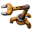Posts: 22
Joined: Fri Apr 01, 2016 11:31 pm
Contact:

### Random Number Generator

Hello everyone,

I am looking for a way to have "something" generate a random number/output. To simplify this the basic concept, I am trying to get to work is having x amount of lamps and have one of them randomly turn on. Any idea how I could achieve this? Inserters do not take a random item from a chest so that does not work, did not figure out a way to do it with Combinators either.

Thank you very much! =)
Join me in my Factorio Let´s Play: https://www.youtube.com/user/Mulematsch

lamesnow
Burner Inserter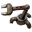Posts: 10
Joined: Sat Apr 02, 2016 5:07 pm
Contact:

### Re: Random Number Generator

Real random number generators are going to be hard (only source of randomness is the player's actions, (and maybe the biters) and you obviously don't want to actually DO something yourself to get random numbers).

This leaves pseudo random number generators (PRNG). They generate numbers that aren't really random, but look random at first sight (This means they're good enough if you want to turn on a random light). An easy example, that is definitely possible (but pretty hard) to implement with combinators, are Linear congruential generators: https://en.wikipedia.org/wiki/Linear_co ... _generator.

orzelek
Smart Inserter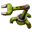Posts: 3654
Joined: Fri Apr 03, 2015 10:20 am
Contact:

### Re: Random Number Generator

You can use built-it random generator from lua - it's multiplayer safe (each peer will get same number on same roll).

ratchetfreak
Filter Inserter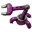Posts: 949
Joined: Sat May 23, 2015 12:10 pm
Contact:

### Re: Random Number Generator

or have a belt with a irregular distribution of items going around and a few inserters taking off and putting back. Add some chests to read what got taken off and use that to adjust the seed.

Then use a typical PRNG implementations implemented in combinators to provide a random number stream.

MrDoomah
Fast Inserter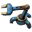Posts: 196
Joined: Mon Jun 01, 2015 1:11 pm
Contact:

### Re: Random Number Generator

If you don't mind using a console command you can use this:

Code: Select all

``````/c
require "defines"
entity = game.player.selected
if entity.type == "constant-combinator" then
script.on_event(defines.events.on_tick,function(event)
if event.tick % 60 == 0 then
if entity.valid then
entity.set_circuit_condition(defines.circuitconditionindex.constant_combinator, {parameters = {{signal = {type = "virtual", name = "signal-0"}, count = math.random(1,100), index = 1}}})
else
script.on_event(defines.events.on_tick,nil)
end
end
end)
end``````
If you hover your mouse over a constant combinator and then use this, that combinator will output a random number between 1 and 100 every second.

If you want to change the range of number, just edit the part in math.random(min, max). If you want an other frequency then you can edit the if event.tick % 60 == 0, where you can change 60 to your preferred frequency (60 is once a second, 120 is once every 2 seconds, 20 is 3x per one second.

I've used this to create a factorio disco for my friends.

DaveMcW
Smart InserterPosts: 3060
Joined: Tue May 13, 2014 11:06 am
Contact:

### Re: Random Number Generator

Here is a pure combinator solution.LCG.png (639.25 KiB) Viewed 5021 times
The first two combinators are a linear congruential generator, specifically the Visual C++ LCG.

The third combinator throws away the last 16 bits.

The fourth constant combinator is the bounds. In this case B=10, so the random numbers will be in the range -9 to 9.

The last five combinators perform a modulo division and return the result. The two + combinators are null operators that just add zero.

ratchetfreak
Filter InserterPosts: 949
Joined: Sat May 23, 2015 12:10 pm
Contact:

### Re: Random Number Generator

DaveMcW wrote:Here is a pure combinator solution.
stuff
If you feed the raw output (before the division by 65k) back into the input then you get a constantly changing output with medium to low strength randomness.

DaveMcW
Smart InserterPosts: 3060
Joined: Tue May 13, 2014 11:06 am
Contact:

### Re: Random Number Generator

Uh... that is how a LCG works. You use the lower bits to increase the randomness of the upper bits.

If you are worried about the constantly changing part, the cycle length is over 30 hours at 60 FPS.

ratchetfreak
Filter InserterPosts: 949
Joined: Sat May 23, 2015 12:10 pm
Contact:

### Re: Random Number Generator

oh I didn't see the red back connection.

Mullematsch
InserterPosts: 22
Joined: Fri Apr 01, 2016 11:31 pm
Contact:

### Re: Random Number Generator

Thank you to everyone for all the input and the nice suggestions. Definitively helped me out a lot and some useful stuff for the future for sure.
DaveMcW wrote:Here is a pure combinator solution.
Random Output
In case anyone is curious what I was working on, I made a little video showcasing my project: https://www.youtube.com/watch?v=CGarFHprh2E. It is nothing special and my first project with combinators but if you have some time to check it out, I would appreciate some feedback on how to improve it.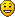Join me in my Factorio Let´s Play: https://www.youtube.com/user/Mulematsch

DaveMcW
Smart InserterPosts: 3060
Joined: Tue May 13, 2014 11:06 am
Contact:

### Re: Random Number Generator

Here is an improved version that should be closer to what you want.LCG2.png (798.82 KiB) Viewed 4557 times
Top Row:
1,2. Memory cell and timer that controls how often the random number refreshes. Currently it is set to one second (60 ticks), but you can change it to whatever you want. One hour = 216000 ticks. Just be sure to set both to the same value.
3. Throw away the 31st bit.
4. Divide the random bits into equal parts.
5. Multiply by -1 to remove negative numbers.

Bottom Row:
1,2. LCG.
3. More constants. A = -2147483648, B = -1, C= 1, you should not change these. D controls the distribution, currently it is set to D=5 which gives a random number from 0 to 4.
4. Divide the random bits into equal parts.
5. If the number is positive, we don't need to multiply by -1. Add +2 (+1 on two wires) to turn the multiplier into a 1.

Mullematsch
InserterPosts: 22
Joined: Fri Apr 01, 2016 11:31 pm
Contact:

### Re: Random Number Generator

DaveMcW wrote:Here is an improved version that should be closer to what you want.
LCG2.png
Top Row:
1,2. Memory cell and timer that controls how often the random number refreshes. Currently it is set to one second (60 ticks), but you can change it to whatever you want. One hour = 216000 ticks. Just be sure to set both to the same value.
3. Throw away the 31st bit.
4. Divide the random bits into equal parts.
5. Multiply by -1 to remove negative numbers.

Bottom Row:
1,2. LCG.
3. More constants. A = -2147483648, B = -1, C= 1, you should not change these. D controls the distribution, currently it is set to D=5 which gives a random number from 0 to 4.
4. Divide the random bits into equal parts.
5. If the number is positive, we don't need to multiply by -1. Add +2 (+1 on two wires) to turn the multiplier into a 1.
Looks awesome. I will have to look into how it does what it does a bit so that I at least kinda get why it is working and not just copy this. Appreciate the help and the explanation of the design as well - thank you!Join me in my Factorio Let´s Play: https://www.youtube.com/user/Mulematsch

zerohourrct
InserterPosts: 26
Joined: Sun Apr 10, 2016 2:57 pm
Contact:

### Re: Random Number Generator

If you just multiplied by the biggest prime number in the set, then divided by the size of the set over the desired combinations, would that be pseudo-random enough? Also super simple. You'd never get zero, so you should get a random number out of -2,-1,1,2 if you divided by half the set size. This may be completely wrong as I have zero knowledge of number theory. The prime number multiplier would be on continuous feedback, output to the divider, and then sampled with a pulsed decider.

EDIT: actually on further thought, due to rounding, you would still get zero. and you'd need to use some weird stuff to return even set sizes. Maybe prime number magic could still work?

DaveMcW
Smart InserterPosts: 3060
Joined: Tue May 13, 2014 11:06 am
Contact:

### Re: Random Number Generator

Yes, the Lehmer RNG does that. But it also requires a prime number set size. The formula is: x = x * 48271 % 2147483647

Unfortunately, modulo is expensive to implement in combinators. A LCG uses two primes and a set size of 2^32, which gets modulo for free using integer overflow.
The formula I used is: x = (x + 2531011) * 214013 % 4294967296

Mensaniac
Manual Inserter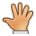Posts: 4
Joined: Sun Apr 17, 2016 11:44 pm
Contact:

### Re: Random Number Generator

Using logic gates, you can build a Linear Feedback Shift Register, which can be used to make a pseudo-random number generator.

https://en.wikipedia.org/wiki/Linear_fe ... t_register

ratchetfreak
Filter InserterPosts: 949
Joined: Sat May 23, 2015 12:10 pm
Contact:

### Re: Random Number Generator

Mensaniac wrote:Using logic gates, you can build a Linear Feedback Shift Register, which can be used to make a pseudo-random number generator.

https://en.wikipedia.org/wiki/Linear_fe ... t_register
But a LCG only needs 2 combinators while a shift register need 1 for each bit (at least)

Serenity
Filter InserterPosts: 635
Joined: Fri Apr 15, 2016 6:16 am
Contact:

### Re: Random Number Generator

They should really add modulo division to the arithmetic combinator. There are also other uses for it

### Who is online

Users browsing this forum: No registered users